x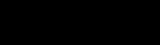DecibelEncyclopedia
The decibel is a logarithmic unit that indicates the ratio of a physical quantity (usually power
Power (physics)
In physics, power is the rate at which energy is transferred, used, or transformed. For example, the rate at which a light bulb transforms electrical energy into heat and light is measured in watts—the more wattage, the more power, or equivalently the more electrical energy is used per unit...

or intensity
Intensity (physics)
In physics, intensity is a measure of the energy flux, averaged over the period of the wave. The word "intensity" here is not synonymous with "strength", "amplitude", or "level", as it sometimes is in colloquial speech...

) relative to a specified or implied reference level. A ratio in decibels is ten times the logarithm to base 10 of the ratio of two power quantities. A decibel is one tenth of a bel, a seldom-used unit.

The decibel is used for a wide variety of measurements in science and engineering
Engineering
Engineering is the discipline, art, skill and profession of acquiring and applying scientific, mathematical, economic, social, and practical knowledge, in order to design and build structures, machines, devices, systems, materials and processes that safely realize improvements to the lives of...

, most prominently in acoustics
Acoustics
Acoustics is the interdisciplinary science that deals with the study of all mechanical waves in gases, liquids, and solids including vibration, sound, ultrasound and infrasound. A scientist who works in the field of acoustics is an acoustician while someone working in the field of acoustics...

, electronics
Electronics
Electronics is the branch of science, engineering and technology that deals with electrical circuits involving active electrical components such as vacuum tubes, transistors, diodes and integrated circuits, and associated passive interconnection technologies...

, and control theory
Control theory
Control theory is an interdisciplinary branch of engineering and mathematics that deals with the behavior of dynamical systems. The desired output of a system is called the reference...

. In electronics, the gain
Gain
In electronics, gain is a measure of the ability of a circuit to increase the power or amplitude of a signal from the input to the output. It is usually defined as the mean ratio of the signal output of a system to the signal input of the same system. It may also be defined on a logarithmic scale,...

s of amplifiers, attenuation
Attenuation
In physics, attenuation is the gradual loss in intensity of any kind of flux through a medium. For instance, sunlight is attenuated by dark glasses, X-rays are attenuated by lead, and light and sound are attenuated by water.In electrical engineering and telecommunications, attenuation affects the...

of signals, and signal-to-noise ratio
Signal-to-noise ratio
Signal-to-noise ratio is a measure used in science and engineering that compares the level of a desired signal to the level of background noise. It is defined as the ratio of signal power to the noise power. A ratio higher than 1:1 indicates more signal than noise...

s are often expressed in decibels. The decibel confers a number of advantages, such as the ability to conveniently represent very large or small numbers, and the ability to carry out multiplication of ratios by simple addition and subtraction.

The decibel symbol is often qualified with a suffix, that indicates which reference quantity or frequency weighting function
A-weighting
A Weighting curve is a graph of a set of factors, that are used to 'weight' measured values of a variable according to their importance in relation to some outcome. The most commonly known example is frequency weighting in sound level measurement where a specific set of weighting curves known as A,...

has been used. For example, dBm
DBm
dBm is an abbreviation for the power ratio in decibels of the measured power referenced to one milliwatt . It is used in radio, microwave and fiber optic networks as a convenient measure of absolute power because of its capability to express both very large and very small values in a short form...

indicates a reference level of one milliwatt, while dBu
DBU
DBU may refer to:* Dansk Boldspil-Union, in English known as the Danish Football Association* dBu, a decibel measurement of voltage* 1,8-Diazabicyclo[5.4.0]undec-7-ene...

is referenced to 0.775 volts RMS
Root mean square
In mathematics, the root mean square , also known as the quadratic mean, is a statistical measure of the magnitude of a varying quantity. It is especially useful when variates are positive and negative, e.g., sinusoids...

.

The definitions of the decibel and bel use base 10 logarithms. The neper
Neper
The neper is a logarithmic unit for ratios of measurements of physical field and power quantities, such as gain and loss of electronic signals. It has the unit symbol Np. The unit's name is derived from the name of John Napier, the inventor of logarithms...

, an alternative logarithmic ratio unit sometimes used, uses the natural logarithm
Natural logarithm
The natural logarithm is the logarithm to the base e, where e is an irrational and transcendental constant approximately equal to 2.718281828...

(base e
E (mathematical constant)
The mathematical constant ' is the unique real number such that the value of the derivative of the function at the point is equal to 1. The function so defined is called the exponential function, and its inverse is the natural logarithm, or logarithm to base...

).

A change in power ratio by a factor of 10 is a 10 dB change. A change in power ratio by a factor of two is approximately a 3 dB change.

## History

The decibel originates from methods used to quantify reductions in audio levels in telephone circuits. These losses were originally measured in units of Miles of Standard Cable (MSC), where 1 MSC corresponded to the loss of power over a 1 mile
Mile
A mile is a unit of length, most commonly 5,280 feet . The mile of 5,280 feet is sometimes called the statute mile or land mile to distinguish it from the nautical mile...

(approximately 1.6 km) length of standard telephone
Telephone
The telephone , colloquially referred to as a phone, is a telecommunications device that transmits and receives sounds, usually the human voice. Telephones are a point-to-point communication system whose most basic function is to allow two people separated by large distances to talk to each other...

cable at a frequency of 5000 radians per second (795.8 Hz), and roughly matched the smallest attenuation detectable to the average listener. Standard telephone cable was defined as "a cable having uniformly distributed resistance of 88 ohms per loop mile and uniformly distributed shunt
Shunt (electrical)
In electronics, a shunt is a device which allows electric current to pass around another point in the circuit. The term is also widely used in photovoltaics to describe an unwanted short circuit between the front and back surface contacts of a solar cell, usually caused by wafer damage.-Defective...

capacitance
Capacitance
In electromagnetism and electronics, capacitance is the ability of a capacitor to store energy in an electric field. Capacitance is also a measure of the amount of electric potential energy stored for a given electric potential. A common form of energy storage device is a parallel-plate capacitor...

" (approximately 19 gauge).

The transmission unit (TU) was devised by engineers of the Bell Telephone Laboratories
Bell Labs
Bell Laboratories is the research and development subsidiary of the French-owned Alcatel-Lucent and previously of the American Telephone & Telegraph Company , half-owned through its Western Electric manufacturing subsidiary.Bell Laboratories operates its...

in the 1920s to replace the MSC. 1 TU was defined as ten times the base-10 logarithm of the ratio of measured power to a reference power level.
The definitions were conveniently chosen such that 1 TU approximately equaled 1 MSC (specifically, 1.056 TU = 1 MSC).
In 1928, the Bell system renamed the TU to the decibel.
Along with the decibel, the Bell System
Bell System
The Bell System was the American Bell Telephone Company and then, subsequently, AT&T led system which provided telephone services to much of the United States and Canada from 1877 to 1984, at various times as a monopoly. In 1984, the company was broken up into separate companies, by a U.S...

defined the bel, the base-10 logarithm of the power ratio, in honor of their founder and telecommunications pioneer Alexander Graham Bell
Alexander Graham Bell
Alexander Graham Bell was an eminent scientist, inventor, engineer and innovator who is credited with inventing the first practical telephone....

.
The bel is seldom used, as the decibel was the proposed working unit.

The naming and early definition of the decibel is described in the NBS
NBS
NBS can stand for:*N-Bromosuccinimide, a chemical reagent*Nagano Broadcasting Systems, a television broadcasting network in Nagano Prefecture, Japan*The NASCAR Busch Series*A Nash bargaining solution, in economics, a solution to a Nash bargaining game...

Standard's Yearbook of 1931:
In April 2003, the International Committee for Weights and Measures
International Committee for Weights and Measures
The Interglobal Committee for Weights and Measures is the English name of the Comité international des poids et mesures . It consists of eighteen persons from Member States of the Metre Convention...

(CIPM) considered a recommendation for the decibel's inclusion in the International System of Units
International System of Units
The International System of Units is the modern form of the metric system and is generally a system of units of measurement devised around seven base units and the convenience of the number ten. The older metric system included several groups of units...

(SI), but decided not to adopt the decibel as an SI unit. However, the decibel is recognized by other international bodies such as the International Electrotechnical Commission
International Electrotechnical Commission
The International Electrotechnical Commission is a non-profit, non-governmental international standards organization that prepares and publishes International Standards for all electrical, electronic and related technologies – collectively known as "electrotechnology"...

(IEC). The IEC permits the use of the decibel with field quantities as well as power and this recommendation is followed by many national standards bodies, such as NIST, which justifies the use of the decibel for voltage ratios.

## Definition

A decibel (dB) is one tenth of a bel (B), i.e. = . The bel is the logarithm of the ratio between two power quantities of 10:1, and for two field quantities in the ratio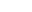. A field quantity is a quantity such as voltage, current, sound pressure, electric field strength, velocity and charge density, the square of which in linear systems is proportional to power. A power quantity is a power or a quantity directly proportional to power, e.g. energy density, acoustic intensity and luminous intensity.

The calculation of the ratio in decibels varies depending on whether the quantity being measured is a power quantity or a field quantity.

Two signals that differ by one decibel have a power ratio of 1.258925411794167 (or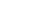) and an amplitude ratio of 1.122018454301963 (or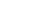).

### Power quantities

When referring to measurements of power
Power (physics)
In physics, power is the rate at which energy is transferred, used, or transformed. For example, the rate at which a light bulb transforms electrical energy into heat and light is measured in watts—the more wattage, the more power, or equivalently the more electrical energy is used per unit...

or intensity
Intensity (physics)
In physics, intensity is a measure of the energy flux, averaged over the period of the wave. The word "intensity" here is not synonymous with "strength", "amplitude", or "level", as it sometimes is in colloquial speech...

, a ratio can be expressed in decibels by evaluating ten times the base-10 logarithm of the ratio of the measured quantity to the reference level. Thus, the ratio of a power value P1 to another power value P0 is represented by LdB, that ratio expressed in decibels, which is calculated using the formula: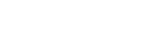The base-10 logarithm of the ratio of the two power levels is the number of bels. The number of decibels is ten times the number of bels (equivalently, a decibel is one-tenth of a bel). P1 and P0 must measure the same type of quantity, and have the same units before calculating the ratio. If P1 = P0 in the above equation, then LdB = 0. If P1 is greater than P0 then LdB is positive; if P1 is less than P0 then LdB is negative.

Rearranging the above equation gives the following formula for P1 in terms of P0 and LdB: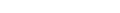.

Since a bel is equal to ten decibels, the corresponding formulae for measurement in bels (LB) are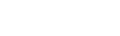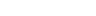.

### Field quantities

When referring to measurements of field amplitude
Amplitude
Amplitude is the magnitude of change in the oscillating variable with each oscillation within an oscillating system. For example, sound waves in air are oscillations in atmospheric pressure and their amplitudes are proportional to the change in pressure during one oscillation...

it is usual to consider the ratio of the squares of A1 (measured amplitude) and A0 (reference amplitude). This is because in most applications power is proportional to the square of amplitude, and it is desirable for the two decibel formulations to give the same result in such typical cases. Thus the following definition is used: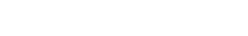This formula is sometimes called the 20 log rule, and similarly the formula for ratios of powers is the 10 log rule, and similarly for other factors. The equivalence of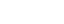and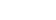is one of the standard properties of logarithms
Logarithm
The logarithm of a number is the exponent by which another fixed value, the base, has to be raised to produce that number. For example, the logarithm of 1000 to base 10 is 3, because 1000 is 10 to the power 3: More generally, if x = by, then y is the logarithm of x to base b, and is written...

.

The formula may be rearranged to give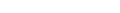Similarly, in electrical circuits
Electronic circuit
An electronic circuit is composed of individual electronic components, such as resistors, transistors, capacitors, inductors and diodes, connected by conductive wires or traces through which electric current can flow...

, dissipated power is typically proportional to the square of voltage
Voltage
Voltage, otherwise known as electrical potential difference or electric tension is the difference in electric potential between two points — or the difference in electric potential energy per unit charge between two points...

or current
Electric current
Electric current is a flow of electric charge through a medium.This charge is typically carried by moving electrons in a conductor such as wire...

when the impedance
Electrical impedance
Electrical impedance, or simply impedance, is the measure of the opposition that an electrical circuit presents to the passage of a current when a voltage is applied. In quantitative terms, it is the complex ratio of the voltage to the current in an alternating current circuit...

is held constant. Taking voltage as an example, this leads to the equation: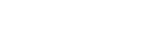where V1 is the voltage being measured, V0 is a specified reference voltage, and GdB is the power gain expressed in decibels. A similar formula holds for current.

### Examples

dB power ratio amplitude ratio
100   10 000 000 000 100 000
90 1 000 000 000 31 620
80 100 000 000 10 000
70 10 000 000 3 162
60 1 000 000 1 000
50 100 000 316 .2
40 10 000 100
30 1 000 31 .62
20 100 10
10 10 3 .162
0 1 1
-10 0 .1 0 .316 2
-20 0 .01 0 .1
-30 0 .001 0 .031 62
-40 0 .000 1 0 .01
-50 0 .000 01 0 .003 162
-60 0 .000 001 0 .001
-70 0 .000 000 1 0 .000 316 2
-80 0 .000 000 01 0 .000 1
-90 0 .000 000 001 0 .000 031 62
-100 0 .000 000 000 1 0 .000 01
An example scale showing power ratios x and amplitude ratios √x and dB equivalents 10 log10 x. It is easier to grasp and compare 2- or 3-digit numbers than to compare up to 10 digits.

All of these examples yield dimensionless answers in dB because they are relative ratios expressed in decibels.
• To calculate the ratio of 1 kW (one kilowatt, or 1000 watts) to 1 W in decibels, use the formula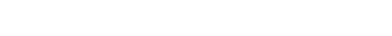• To calculate the ratio of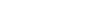to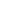in decibels, use the formula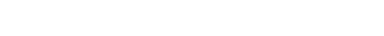Notice that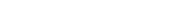, illustrating the consequence from the definitions above that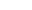has the same value,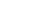, regardless of whether it is obtained with the 10-log or 20-log rules; provided that in the specific system being considered power ratios are equal to amplitude ratios squared.
• To calculate the ratio of 1 mW (one milliwatt) to 10 W in decibels, use the formula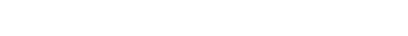• To find the power ratio corresponding to a 3 dB change in level, use the formula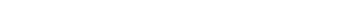A change in power ratio by a factor of 10 is a 10 dB change. A change in power ratio by a factor of two is approximately a 3 dB change. More precisely, the factor is 103/10, or 1.9953, about 0.24% different from exactly 2. Similarly, an increase of 3 dB implies an increase in voltage by a factor of approximately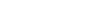, or about 1.41, an increase of 6 dB corresponds to approximately four times the power and twice the voltage, and so on. In exact terms the power ratio is 106/10, or about 3.9811, a relative error of about 0.5%.

## Merits

The use of the decibel has a number of merits:
• The decibel's logarithm
Logarithm
The logarithm of a number is the exponent by which another fixed value, the base, has to be raised to produce that number. For example, the logarithm of 1000 to base 10 is 3, because 1000 is 10 to the power 3: More generally, if x = by, then y is the logarithm of x to base b, and is written...

ic nature means that a very large range of ratios can be represented by a convenient number, in a similar manner to scientific notation
Scientific notation
Scientific notation is a way of writing numbers that are too large or too small to be conveniently written in standard decimal notation. Scientific notation has a number of useful properties and is commonly used in calculators and by scientists, mathematicians, doctors, and engineers.In scientific...

. This allows one to clearly visualize huge changes of some quantity. (See Bode Plot
Bode plot
A Bode plot is a graph of the transfer function of a linear, time-invariant system versus frequency, plotted with a log-frequency axis, to show the system's frequency response...

and half logarithm graph.)
• The mathematical properties of logarithms mean that the overall decibel gain of a multi-component system (such as consecutive amplifiers) can be calculated simply by summing the decibel gains of the individual components, rather than needing to multiply amplification factors. Essentially this is because log(A × B × C × ...) = log(A) + log(B) + log(C) + ...
• The human perception of the intensity of, for example, sound or light, is more nearly proportional to the logarithm of intensity than to the intensity itself, per the Weber–Fechner law
Weber–Fechner law
The Weber–Fechner law is a confusing term, because it combines two different laws. Some authors use the term to mean Weber's law, and others Fechner's law. Fechner himself added confusion to the literature by calling his own law Weber's law...

, so the dB scale can be useful to describe perceptual levels or level differences.

### Acoustics

The decibel is commonly used in acoustics
Acoustics
Acoustics is the interdisciplinary science that deals with the study of all mechanical waves in gases, liquids, and solids including vibration, sound, ultrasound and infrasound. A scientist who works in the field of acoustics is an acoustician while someone working in the field of acoustics...

to quantify sound
Sound
Sound is a mechanical wave that is an oscillation of pressure transmitted through a solid, liquid, or gas, composed of frequencies within the range of hearing and of a level sufficiently strong to be heard, or the sensation stimulated in organs of hearing by such vibrations.-Propagation of...

levels relative to a 0 dB reference which has been defined as a sound pressure level of .0002 microbar. The reference level is set at the typical threshold of perception of an average human and there are common comparisons used to illustrate different levels of sound pressure. As with other decibel figures, normally the ratio expressed is a power ratio (rather than a pressure ratio).

The human ear has a large dynamic range in audio perception. The ratio of the sound intensity that causes permanent damage during short exposure to the quietest sound that the ear can hear is greater than or equal to 1 trillion. Such large measurement ranges are conveniently expressed in logarithmic units: the base-10 logarithm of one trillion (1012) is 12, which is expressed as an audio level of 120 dB. Since the human ear is not equally sensitive to all sound frequencies, noise levels at maximum human sensitivity—somewhere between 2 and 4 kHz
Hertz
The hertz is the SI unit of frequency defined as the number of cycles per second of a periodic phenomenon. One of its most common uses is the description of the sine wave, particularly those used in radio and audio applications....

—are factored more heavily into some measurements using frequency weighting. (See also Stevens' power law
Stevens' power law
Stevens' power law is a proposed relationship between the magnitude of a physical stimulus and its perceived intensity or strength. It is often considered to supersede the Weber–Fechner law on the basis that it describes a wider range of sensations, although critics argue that the validity of the...

.)

### Electronics

In electronics, the decibel is often used to express power or amplitude ratios (gain
Gain
In electronics, gain is a measure of the ability of a circuit to increase the power or amplitude of a signal from the input to the output. It is usually defined as the mean ratio of the signal output of a system to the signal input of the same system. It may also be defined on a logarithmic scale,...

s), in preference to arithmetic
Arithmetic
Arithmetic or arithmetics is the oldest and most elementary branch of mathematics, used by almost everyone, for tasks ranging from simple day-to-day counting to advanced science and business calculations. It involves the study of quantity, especially as the result of combining numbers...

ratios or percentages. One advantage is that the total decibel gain of a series of components (such as amplifier
Amplifier
Generally, an amplifier or simply amp, is a device for increasing the power of a signal.In popular use, the term usually describes an electronic amplifier, in which the input "signal" is usually a voltage or a current. In audio applications, amplifiers drive the loudspeakers used in PA systems to...

s and attenuators) can be calculated simply by summing the decibel gains of the individual components. Similarly, in telecommunications, decibels denote signal gain or loss from a transmitter to a receiver through some medium (free space, waveguide
Waveguide
A waveguide is a structure which guides waves, such as electromagnetic waves or sound waves. There are different types of waveguides for each type of wave...

, coax, fiber optics, etc.) using a link budget
A link budget is the accounting of all of the gains and losses from the transmitter, through the medium to the receiver in a telecommunication system. It accounts for the attenuation of...

.

The decibel unit can also be combined with a suffix to create an absolute unit of electric power. For example, it can be combined with "m" for "milliwatt" to produce the "dBm
DBm
dBm is an abbreviation for the power ratio in decibels of the measured power referenced to one milliwatt . It is used in radio, microwave and fiber optic networks as a convenient measure of absolute power because of its capability to express both very large and very small values in a short form...

". Zero dBm equals one milliwatt, and 1 dBm is one decibel greater (about 1.259 mW).

In professional audio, a popular unit is the dBu (see below for all the units). The "u" stands for "unloaded", and was probably chosen to be similar to lowercase "v", as dBv was the older name for the same thing. It was changed to avoid confusion with dBV. This unit (dBu) is an RMS
Root mean square
In mathematics, the root mean square , also known as the quadratic mean, is a statistical measure of the magnitude of a varying quantity. It is especially useful when variates are positive and negative, e.g., sinusoids...

measurement of voltage which uses as its reference 0.775 VRMS. Chosen for historical reasons, it is the voltage level which delivers 1 mW of power in a 600 ohm resistor, which used to be the standard reference impedance in telephone audio circuits.

### Optics

An optical link is a communications link that consists of a single end-to-end optical circuit. A cable of optical fiber, possibly concatenated into a dark fiber link, is the simplest form of an optical link....

, if a known amount of optical
Optics
Optics is the branch of physics which involves the behavior and properties of light, including its interactions with matter and the construction of instruments that use or detect it. Optics usually describes the behavior of visible, ultraviolet, and infrared light...

power, in dBm
DBm
dBm is an abbreviation for the power ratio in decibels of the measured power referenced to one milliwatt . It is used in radio, microwave and fiber optic networks as a convenient measure of absolute power because of its capability to express both very large and very small values in a short form...

(referenced to 1 mW), is launched into a fiber
Optical fiber
An optical fiber is a flexible, transparent fiber made of a pure glass not much wider than a human hair. It functions as a waveguide, or "light pipe", to transmit light between the two ends of the fiber. The field of applied science and engineering concerned with the design and application of...

, and the losses, in dB (decibels), of each electronic component
Electronic component
An electronic component is a basic electronic element and may be available in a discrete form having two or more electrical terminals . These are intended to be connected together, usually by soldering to a printed circuit board, in order to create an electronic circuit with a particular function...

(e.g., connectors, splices, and lengths of fiber) are known, the overall link loss may be quickly calculated by addition and subtraction of decibel quantities.

In spectrometry and optics, the blocking unit used to measure optical density is equivalent to −1 B.

### Video and digital imaging

In connection with video and digital image sensor
Image sensor
An image sensor is a device that converts an optical image into an electronic signal. It is used mostly in digital cameras and other imaging devices...

s, decibels generally represent ratios of video voltages or digitized light levels, using 20 log of the ratio, even when the represented optical power is directly proportional to the voltage or level, not to its square, as in a CCD imager where response voltage is linear in intensity.
Thus, a camera signal-to-noise ratio
Signal-to-noise ratio
Signal-to-noise ratio is a measure used in science and engineering that compares the level of a desired signal to the level of background noise. It is defined as the ratio of signal power to the noise power. A ratio higher than 1:1 indicates more signal than noise...

or dynamic range
Dynamic range
Dynamic range, abbreviated DR or DNR, is the ratio between the largest and smallest possible values of a changeable quantity, such as in sound and light. It is measured as a ratio, or as a base-10 or base-2 logarithmic value.-Dynamic range and human perception:The human senses of sight and...

of 40 dB represents a power ratio of 100:1 between signal power and noise power, not 10,000:1.
Sometimes the 20 log ratio definition is applied to electron counts or photon counts directly, which are proportional to intensity without the need consider whether the voltage response is linear.

However, as mentioned above, the 10 log intensity convention prevails more generally in physical optics, including fiber optics, so the terminology can become murky between the conventions of digital photographic technology and physics. Most commonly, quantities called "dynamic range" or "signal-to-noise" (of the camera) would be specified in 20 log dBs, but in related contexts (e.g. attenuation, gain, intensifier SNR, or rejection ratio) the term should be interpreted cautiously, as confusion of the two units can result in very large misunderstandings of the value.

Photographers also often use an alternative base-2 log unit, the f-stop, and in software contexts these image level ratios, particularly dynamic range, are often loosely referred to by the number of bits needed to represent the quantity, such that 60 dB (digital photographic) is roughly equal to 10 f-stops or 10 bits, since 103 is nearly equal to 210.

## Suffixes and reference levels

Suffixes are commonly attached to the basic dB unit in order to indicate the reference level against which the decibel measurement is taken. For example, dBm indicates power measurement relative to 1 milliwatt.

In cases such as this, where the numerical value of the reference is explicitly and exactly stated, the decibel measurement is called an "absolute" measurement, in the sense that the exact value of the measured quantity can be recovered using the formula given earlier. If the numerical value of the reference is not explicitly stated, as in the dB gain of an amplifier, then the decibel measurement is purely relative.

The SI does not permit attaching qualifiers to units, whether as suffix or prefix, other than standard SI prefix
SI prefix
The International System of Units specifies a set of unit prefixes known as SI prefixes or metric prefixes. An SI prefix is a name that precedes a basic unit of measure to indicate a decadic multiple or fraction of the unit. Each prefix has a unique symbol that is prepended to the unit symbol...

es. Therefore, even though the decibel is accepted for use alongside SI units, the practice of attaching a suffix to the basic dB unit, forming compound units such as dBm, dBu, dBA, etc., is not. However, outside of documents adhering to SI units, the practice is very common as illustrated by the following examples.

### Voltage

Since the decibel is defined with respect to power, not amplitude, conversions of voltage ratios to decibels must square the amplitude, as discussed above.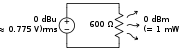dBV
dB(1 VRMS
Root mean square
In mathematics, the root mean square , also known as the quadratic mean, is a statistical measure of the magnitude of a varying quantity. It is especially useful when variates are positive and negative, e.g., sinusoids...

) – volt
Volt
The volt is the SI derived unit for electric potential, electric potential difference, and electromotive force. The volt is named in honor of the Italian physicist Alessandro Volta , who invented the voltaic pile, possibly the first chemical battery.- Definition :A single volt is defined as the...

age relative to 1 volt, regardless of impedance.

dBu or dBv
dB(0.775 VRMS
Root mean square
In mathematics, the root mean square , also known as the quadratic mean, is a statistical measure of the magnitude of a varying quantity. It is especially useful when variates are positive and negative, e.g., sinusoids...

) – volt
Volt
The volt is the SI derived unit for electric potential, electric potential difference, and electromotive force. The volt is named in honor of the Italian physicist Alessandro Volta , who invented the voltaic pile, possibly the first chemical battery.- Definition :A single volt is defined as the...

age relative to 0.775 volts. Originally dBv, it was changed to dBu to avoid confusion with dBV. The "v" comes from "volt", while "u" comes from "unloaded". dBu can be used regardless of impedance, but is derived from a 600 Ω load dissipating 0 dBm (1 mW). Reference voltage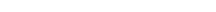In professional audio
Professional audio
Professional audio, also 'pro audio', refers to both an activity and a type of audio equipment. Typically it encompasses the production or reproduction of sound for an audience, by individuals who do such work as an occupation like live event support, using sound reinforcement systems designed for...

, equipment may be calibrated to indicate a "0" on the VU meter
VU meter
A VU meter is often included in audio equipment to display a signal level in Volume Units; the device is sometimes also called volume indicator ....

s some finite time after a signal has been applied at an amplitude of . Consumer equipment will more often use a much lower "nominal" signal level of . Therefore, many devices offer dual voltage operation (with different gain or "trim" settings) for interoperability reasons. A switch or adjustment that covers at least the range between and is common in professional equipment.

dBmV
dB(1 mVRMS
Root mean square
In mathematics, the root mean square , also known as the quadratic mean, is a statistical measure of the magnitude of a varying quantity. It is especially useful when variates are positive and negative, e.g., sinusoids...

) – volt
Volt
The volt is the SI derived unit for electric potential, electric potential difference, and electromotive force. The volt is named in honor of the Italian physicist Alessandro Volta , who invented the voltaic pile, possibly the first chemical battery.- Definition :A single volt is defined as the...

age relative to 1 millivolt across 75 Ω. Widely used in cable television
Cable television
Cable television is a system of providing television programs to consumers via radio frequency signals transmitted to televisions through coaxial cables or digital light pulses through fixed optical fibers located on the subscriber's property, much like the over-the-air method used in traditional...

networks, where the nominal strength of a single TV signal at the receiver terminals is about 0 dBmV. Cable TV uses 75 Ω coaxial cable, so 0 dBmV corresponds to −78.75 dBW (−48.75 dBm) or ~13 nW.

dBμV or dBuV
dB(1 μVRMS
Root mean square
In mathematics, the root mean square , also known as the quadratic mean, is a statistical measure of the magnitude of a varying quantity. It is especially useful when variates are positive and negative, e.g., sinusoids...

) – volt
Volt
The volt is the SI derived unit for electric potential, electric potential difference, and electromotive force. The volt is named in honor of the Italian physicist Alessandro Volta , who invented the voltaic pile, possibly the first chemical battery.- Definition :A single volt is defined as the...

age relative to 1 microvolt. Widely used in television and aerial amplifier specifications. 60 dBμV = 0 dBmV.

### Acoustics

Probably the most common usage of "decibels" in reference to sound loudness is dB SPL, sound pressure level referenced to the nominal threshold of human hearing:

dB(SPL)
dB (sound pressure level) – for sound in air and other gases, relative to 20 micropascals (μPa) = 2×10−5 Pa, the quietest sound a human can hear. This is roughly the sound of a mosquito flying 3 meters away. This is often abbreviated to just "dB", which gives some the erroneous notion that "dB" is an absolute unit by itself. For sound in water
Underwater acoustics
Underwater acoustics is the study of the propagation of sound in water and the interaction of the mechanical waves that constitute sound with the water and its boundaries. The water may be in the ocean, a lake or a tank. Typical frequencies associated with underwater acoustics are between 10 Hz and...

and other liquids, a reference pressure of 1 μPa is used.

One Pascal is equal to 94 dB(SPL). This level is used to specify microphone sensitivity. For example, a typical microphone may put out 20 mV at one pascal. For other sound pressure levels, the output voltage can be computed from this basis, except that noise and distortion will affect the extreme levels.

dB(PA)
dB – relative to 1 Pa, often used in telecommunications.

dB SIL
dB sound intensity level – relative to 10−12 W/m2, which is roughly the threshold of human hearing in air.

dB SWL
dB sound power level – relative to 10−12 W.

dB(A), dB(B), and dB(C)
These symbols are often used to denote the use of different weighting filter
Weighting filter
A weighting filter is used to emphasise or suppress some aspects of a phenomenon compared to others, for measurement or other purposes.- Audio applications :...

s, used to approximate the human ear's response
Stimulus (psychology)
In psychology, stimuli are energy patterns which are registered by the senses. In behaviorism and related stimulus–response theories, stimuli constitute the basis for behavior, whereas in perceptual psychology they constitute the basis for perception.In the second half of the 19th century, the...

to sound, although the measurement is still in dB (SPL). These measurements usually refer to noise and noisome effects on humans and animals, and are in widespread use in the industry with regard to noise control issues, regulations and environmental standards. Other variations that may be seen are dBA or dBA
A-weighting
A Weighting curve is a graph of a set of factors, that are used to 'weight' measured values of a variable according to their importance in relation to some outcome. The most commonly known example is frequency weighting in sound level measurement where a specific set of weighting curves known as A,...

. According to ANSI standards, the preferred usage is to write LA = x dB. Nevertheless, the units dBA and dB(A) are still commonly used as a shorthand for A-weighted measurements. Compare dBc
DBc
dBc is the power ratio of a signal to a carrier signal, expressed in decibels. For example, phase noise is expressed in dBc/Hz at a given frequency offset from the carrier...

, used in telecommunications.

dB HL or dB hearing level is used in audiogram
Audiogram
An audiogram is a standard way of representing a person's hearing loss . Most audiograms cover the limited range 100 Hz to 8000 Hz which is most important for clear understanding of speech, and they plot the threshold of hearing relative to a standardised curve that represents 'normal'...

s as a measure of hearing loss. The reference level varies with frequency according to a minimum audibility curve
Minimum audibility curve
Minimum audibility curve is a standardised graph of the threshold of hearing versus frequency for an average human, and is used as the reference level when measuring hearing loss with an audiometer as shown on an audiogram....

as defined in ANSI and other standards, such that the resulting audiogram shows deviation from what is regarded as 'normal' hearing.

dB Q is sometimes used to denote weighted noise level, commonly using the ITU-R 468 noise weighting
ITU-R 468 noise weighting
ITU-R 468 is a standard relating to noise measurement, widely used when measuring noise in audio systems. The standard defines a weighting filter curve, together with a quasi-peak rectifier having special characteristics as defined by specified tone-burst tests...

### Audio electronics

dBm
DBm
dBm is an abbreviation for the power ratio in decibels of the measured power referenced to one milliwatt . It is used in radio, microwave and fiber optic networks as a convenient measure of absolute power because of its capability to express both very large and very small values in a short form...

dB(mW) – power relative to 1 milliwatt. No reference impedance is assumed, although 600 ohms is common in audio equipment.

dBFS
DBFS
Decibels relative to full scale, commonly abbreviated dBFS, measures decibel amplitude levels in digital systems such as pulse-code modulation which have a defined maximum available peak level....

dB(full scale
Full scale
In electronics and signal processing, full scale or full code represents the maximum amplitude a system can present.-Electronics and signal processing:...

) – the amplitude
Amplitude
Amplitude is the magnitude of change in the oscillating variable with each oscillation within an oscillating system. For example, sound waves in air are oscillations in atmospheric pressure and their amplitudes are proportional to the change in pressure during one oscillation...

of a signal compared with the maximum which a device can handle before clipping
Clipping (signal processing)
Clipping is a form of distortion that limits a signal once it exceeds a threshold. Clipping may occur when a signal is recorded by a sensor that has constraints on the range of data it can measure, it can occur when a signal is digitized, or it can occur any other time an analog or digital signal...

occurs. Full-scale may be defined as the power level of a full-scale sinusoid or alternatively a full-scale square wave
Square wave
A square wave is a kind of non-sinusoidal waveform, most typically encountered in electronics and signal processing. An ideal square wave alternates regularly and instantaneously between two levels...

.

dBTP
dB(true peak) - peak amplitude of a signal compared with the maximum which a device can handle before clipping occurs. In digital systems, 0 dBTP would equal the highest level (number) the processor is capable of representing. Measured values are always negative or zero, since they are less than or equal to full-scale.

dBZ
DBZ (meteorology)
dBZ stands for decibels of Z. It is a meteorological measure of equivalent reflectivity of a radar signal reflected off a remote object. The reference level for Z is 1 mm6 m−3, which is equal to 1 μm3...

dB(Z) – energy of reflectivity (weather radar), related to the amount of transmitted power returned to the radar receiver; the reference level for Z is 1 mm6 m−3. Values above 15–20 dBZ usually indicate falling precipitation.

dBsm
dBsm – decibel measure of the radar cross section
Radar cross section is a measure of how detectable an object is with a radar. A larger RCS indicates that an object is more easily detected.An object reflects a limited amount of radar energy...

(RCS) of a target relative one square meter. The power reflected by the target is proportional to its RCS. "Stealth" aircraft and insects have negative RCS measured in dBsm, large flat plates or non-stealthy aircraft have positive values.

### Radio power, energy, and field strength

dBc
DBc
dBc is the power ratio of a signal to a carrier signal, expressed in decibels. For example, phase noise is expressed in dBc/Hz at a given frequency offset from the carrier...

dBc – relative to carrier—in telecommunication
Telecommunication
Telecommunication is the transmission of information over significant distances to communicate. In earlier times, telecommunications involved the use of visual signals, such as beacons, smoke signals, semaphore telegraphs, signal flags, and optical heliographs, or audio messages via coded...

s, this indicates the relative levels of noise or sideband peak power, compared with the carrier power. Compare dBC, used in acoustics.

dBJ
dB(J) – energy relative to 1 joule
Joule
The joule ; symbol J) is a derived unit of energy or work in the International System of Units. It is equal to the energy expended in applying a force of one newton through a distance of one metre , or in passing an electric current of one ampere through a resistance of one ohm for one second...

. 1 joule = 1 watt per hertz, so power spectral density can be expressed in dBJ.

dBm
DBm
dBm is an abbreviation for the power ratio in decibels of the measured power referenced to one milliwatt . It is used in radio, microwave and fiber optic networks as a convenient measure of absolute power because of its capability to express both very large and very small values in a short form...

dB(mW) – power relative to 1 milliwatt. When used in the radio field, the dB is usually referenced to a 50 ohm load, with the resultant voltage being 0.224 volts. There are times when spec sheets may show the voltage & power level e.g. −120 dBm = 0.224 microvolts.

dBμV/m or dBuV/m
dB(μV/m) – electric field strength relative to 1 microvolt per meter. Often used to specify the signal strength from a television
Television
Television is a telecommunication medium for transmitting and receiving moving images that can be monochrome or colored, with accompanying sound...

at a receiving site (the signal measured at the antenna output will be in dBμV).

dBf
dB(fW) – power relative to 1 femtowatt.

dBW
dB(W) – power relative to 1 watt
Watt
The watt is a derived unit of power in the International System of Units , named after the Scottish engineer James Watt . The unit, defined as one joule per second, measures the rate of energy conversion.-Definition:...

.

dBk
dB(kW) – power relative to 1 kilowatt.

### Antenna measurements

dBi
dB(isotropic) – the forward gain of an antenna
Antenna gain
In electromagnetics, an antenna's power gain or simply gain is a key performance figure which combines the antenna's directivity and electrical efficiency. As a transmitting antenna, the figure describes how well the antenna converts input power into radio waves headed in a specified direction...

compared with the hypothetical isotropic antenna, which uniformly distributes energy in all directions. Linear polarization
Linear polarization
In electrodynamics, linear polarization or plane polarization of electromagnetic radiation is a confinement of the electric field vector or magnetic field vector to a given plane along the direction of propagation...

of the EM field is assumed unless noted otherwise.

dBd
dB(dipole) – the forward gain of an antenna compared with a half-wave dipole antenna
Dipole antenna
A dipole antenna is a radio antenna that can be made of a simple wire, with a center-fed driven element. It consists of two metal conductors of rod or wire, oriented parallel and collinear with each other , with a small space between them. The radio frequency voltage is applied to the antenna at...

. 0 dBd = 2.15 dBi

dBiC
dB(isotropic circular) – the forward gain of an antenna compared to a circularly polarized
Circular polarization
In electrodynamics, circular polarization of an electromagnetic wave is a polarization in which the electric field of the passing wave does not change strength but only changes direction in a rotary type manner....

isotropic antenna. There is no fixed conversion rule between dBiC and dBi, as it depends on the receiving antenna and the field polarization.

dBq
dB(quarterwave) – the forward gain of an antenna compared to a quarter wavelength whip. Rarely used, except in some marketing material. 0 dBq = −0.85 dBi

### Other measurements

dB-Hz
dB(hertz
Hertz
The hertz is the SI unit of frequency defined as the number of cycles per second of a periodic phenomenon. One of its most common uses is the description of the sine wave, particularly those used in radio and audio applications....

) – bandwidth relative to 1 Hz. E.g., 20 dB-Hz corresponds to a bandwidth of 100 Hz. Commonly used in link budget
A link budget is the accounting of all of the gains and losses from the transmitter, through the medium to the receiver in a telecommunication system. It accounts for the attenuation of...

calculations. Also used in carrier-to-noise-density ratio
In satellite communications, carrier-to-receiver noise density is the ratio of the received carrier power to the receiver noise power density.The carrier-to-receiver noise density ratio is usually expressed in dBHz....

(not to be confused with carrier-to-noise ratio
Carrier-to-noise ratio
In telecommunications, the carrier-to-noise ratio, often written CNR or C/N, is the signal-to-noise ratio of a modulated signal. The term is used to distinguish the CNR of the radio frequency passband signal from the SNR of an analogue base band message signal after demodulation, for example an...

, in dB).

dBov or dBO

) – the amplitude
Amplitude
Amplitude is the magnitude of change in the oscillating variable with each oscillation within an oscillating system. For example, sound waves in air are oscillations in atmospheric pressure and their amplitudes are proportional to the change in pressure during one oscillation...

of a signal (usually audio) compared with the maximum which a device can handle before clipping
Clipping (signal processing)
Clipping is a form of distortion that limits a signal once it exceeds a threshold. Clipping may occur when a signal is recorded by a sensor that has constraints on the range of data it can measure, it can occur when a signal is digitized, or it can occur any other time an analog or digital signal...

occurs. Similar to dBFS, but also applicable to analog systems.

dBr
dB(relative) – simply a relative difference from something else, which is made apparent in context. The difference of a filter's response to nominal levels, for instance.

dBrn
DBrn
The symbol dBrn or dB is an abbreviation for decibels above reference noise.Weighted noise power in dB is referred to 1.0 picowatt. Thus, 0 dBrn = -90 dBm...

dB above reference noise
Reference noise
In telecommunication, reference noise is the magnitude of circuit noise chosen as a reference for measurement.Many different levels with a number of different weightings are in current use, and care must be taken to ensure that the proper parameters are stated.Specific ones include: dBa, dBa, dBa,...

DBrnC
dBrnC represents an audio level measurement, typically in a telephone circuit, relative to the circuit noise level, with the measurement of this level frequency-weighted by a standard C-message weighting filter. The C-message weighting filter was chiefly used in North America. The Psophometric...

.

• Apparent magnitude
Apparent magnitude
The apparent magnitude of a celestial body is a measure of its brightness as seen by an observer on Earth, adjusted to the value it would have in the absence of the atmosphere...

• Cent
Cent (music)
The cent is a logarithmic unit of measure used for musical intervals. Twelve-tone equal temperament divides the octave into 12 semitones of 100 cents each...

in music
• dB drag racing
DB drag racing
dB drag racing is a competition rewarding the person who can produce the loudest sound inside a vehicle. The "dB" means decibels of sound pressure level . In these competitions, Sound Pressure Level of 155 dB can be reached, and it is not unheard of to see more than 160 dB as well...

• Equal-loudness contour
Equal-loudness contour
An equal-loudness contour is a measure of sound pressure , over the frequency spectrum, for which a listener perceives a constant loudness when presented with pure steady tones. The unit of measurement for loudness levels is the phon, and is arrived at by reference to equal-loudness contours...

• Noise (environmental)
• Phon
Phon
The phon was proposed in DIN 45631 and ISO 532 B as a unit of perceived loudness level LN for pure tones by S. S. Stevens.-Definition:The purpose of the phon scale is to compensate for the effect of frequency on the perceived loudness of tones...

• Richter magnitude scale
Richter magnitude scale
The expression Richter magnitude scale refers to a number of ways to assign a single number to quantify the energy contained in an earthquake....

• Signal noise
• Weighting filter
Weighting filter
A weighting filter is used to emphasise or suppress some aspects of a phenomenon compared to others, for measurement or other purposes.- Audio applications :...

—discussion of dBA